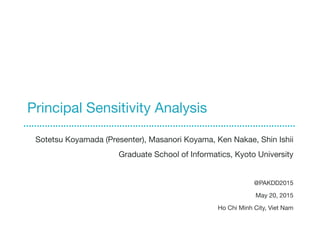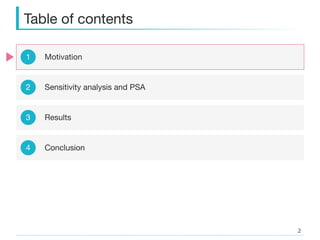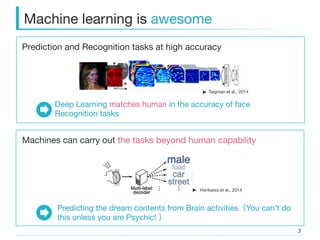Successfully reported this slideshow.

# Principal Sensitivity Analysis

3

Share×
1 of 28
1 of 28

# Principal Sensitivity Analysis

3

Share

#### Description

Sotetsu Koyamada (Presenter), Masanori Koyama, Ken Nakae, Shin Ishii
Graduate School of Informatics, Kyoto University

[Abstract]
We present a novel algorithm (Principal Sensitivity Analysis; PSA) to analyze the knowledge of the classifier obtained from supervised machine learning techniques. In particular, we define principal sensitivity map (PSM) as the direction on the input space to which the trained classifier is most sensitive, and use analogously defined k-th PSM to define a basis for the input space. We train neural networks with artificial data and real data, and apply the algorithm to the obtained supervised classifiers. We then visualize the PSMs to demonstrate the PSA’s ability to decompose the knowledge acquired by the trained classifiers.

[Keywords]
Sensitivity analysis Sensitivity map PCA Dark knowledge Knowledge decomposition

@PAKDD2015
May 20, 2015
Ho Chi Minh City, Viet Namﳟ

#### Transcript

1. 1. Principal Sensitivity Analysis Sotetsu Koyamada (Presenter), Masanori Koyama, Ken Nakae, Shin Ishii Graduate School of Informatics, Kyoto University @PAKDD2015 May 20, 2015 Ho Chi Minh City, Viet Nam
3. 3. Prediction and Recognition tasks at high accuracy Machine learning is awesome Horikawa et al., 2014 Taigman et al., 2014 Machines can carry out the tasks beyond human capability Deep Learning matches human in the accuracy of face Recognition tasks Predicting the dream contents from Brain activities You can’t do this unless you are Psychic!
4. 4. How can the machines carry out the tasks beyond our capability? How can we learn the machine’s “secret” knowledge? In the process of training, machines must have learned the knowledge not in our natural scope
5. 5. Machine is a black box Neural Networks, Nonlinear kernel SVM, … Input Classiﬁcation result ?
6. 6. Visualizing the knowledge of Linear Model The knowledge of the linear classiﬁers like Logistic Regression are expressible in terms of weight parameters w = (w1,…, wd) Classiﬁer Input x = (x1,…, xd) Classiﬁcation labels: {0, 1} wi : weight parameter b: bias parameter σ : sigmoid activation function Meaning of wi = Importance of i-th input dimension within machine’s knowledge
7. 7. It is extremely diﬃcult to make sense out of weight parameters in Neural Networks (nonlinear composition of logistic regressions) Visualizing the knowledge of non Linear Model Our proposal We shall directly analyze the behavior of f in the input space! Meaning of wij (k) = ??????? h: nonlinear activation function
9. 9. Sensitivity analysis Sensitivity analysis compute the sensitivity of f with respect to i-th input dimension Def. Sensitivity with respect to i-th input dimension Note Def. Sensitivity map Zurada et al., 1994, 97, Kjems et al., 2002 q: true distribution of x In the case of linear model (e.g. logistic regression)
10. 10. c.f. Sensitivity with respect to i-th input dimension PSM: Principal Sensitivity Map Deﬁne the directional sensitivity in the arbitrary direction and seek the the direction to which the machine is most sensitive Def. (1st) Principal Sensitivity Map Def. Directional sensitivity in the direction v Recall ei: standard basis of
11. 11. PSA: Principal Sensitivity Analysis Deﬁne the kernel metric K as: Def. (1st) Principal Sensitivity Map (PSM) 1st PSM is the dominant eigen vector of K! When K is covariance matrix, 1st PSM is same as the 1st PC Recall PSA vs PCA
12. 12. PSA: Principal Sensitivity Analysis Deﬁne the kernel metric K as: Def. (1st) Principal Sensitivity Map (PSM) 1st PSM is the dominant eigen vector of K! k-th PSM is the k-th dominant eigen vector of K! Def. (k-th) Principal Sensitivity Map (PSM) When K is covariance matrix, 1st PSM is same as the 1st PC Recall k-th PSM := analogue of k-th PC PSA vs PCA
13. 13. Table of contents 2 3 4 Sensitivity analysis and PSA Numerical Experiments Conclusion 1 Motivation
14. 14. Digit classiﬁcation !  Artiﬁcial Data Each pixel have the same meaning !  Classiﬁer –  Neural Network (one hidden layer) –  Error percentage: 0.36% –  We applied the PSA to the log of each output from NN (b) Noisy samples (a) Templates c = 0, …, 9 c = 0, ….9
15. 15. Strength of PSA (relatively signed map) (b) 1st PSMs (proposed) (a) (Conventional) sensitivity maps
16. 16. visualize the values of Strength of PSA (relatively signed map) (Conventional) sensitivity map cannot distinguish the set of the edges whose presence characterizes the class 1 and The set of the edges whose absence characterizes the class 1 On the other hand, 1st PSM (proposed) can! (b) 1st PSMs (proposed) (a) (Conventional) sensitivity maps visualize the values of
17. 17. Strength of the PSA (sub PSM) PSMs of f9 (c = 9) What is the meaning of sub PSMs (Same as the previous slide)
18. 18. Strength of the PSA (sub PSM) PSMs (c = 9) By deﬁnition, globally important knowledge Perhaps locally important knowledge?
19. 19. Local Sensitivity Def. Local sensitivity in the region A sA(v) := EA ∂fc(x) ∂v 2 Expectation over the region A
20. 20. Local Sensitivity Measure of the contribution of k-th PSM in the classiﬁcation of class c in the subset A Def. Local sensitivity in the region A sk A := sA(vk) k-th PSM sA(v) := EA ∂fc(x) ∂v 2 Def. Local sensitivity in the direction of k-th PSM
21. 21. Local Sensitivity Measure of the contribution of k-th PSM in the classiﬁcation of class c in the subset A Def. Local sensitivity in the region A c = 9, k = 1, A = A(9,4) := set of all the samples of the classes 9 and 4 SA k is The contribution of 1st PSM in the classiﬁcation of 9 in the data containing class 9 and 4 = The contribution of 1st PSM in distinguishing 9 from 4. sk A := sA(vk) k-th PSM sA(v) := EA ∂fc(x) ∂v 2 Def. Local sensitivity in the direction of k-th PSM Example
22. 22. Strength of the PSA (sub PSM) Let’s look at what the knowledge of f9 is doing in distinguishing the pairs of classes (class 9 vs the other class) Local sensitivity of k-th PSM of f9 on the subdata containing class 9 and class c’ ( = A(9, c’) ) c = 9
23. 23. Strength of the PSA (sub PSM) Let’s look at what the knowledge of f9 is doing in distinguishing the pairs of classes (class 9 vs the other class) Local sensitivity of k-th PSM of f9 on the subdata containing class 9 and class c’ c = 9 Example: c = 9, c’ = 4, k = 1 Recall This indicates the contribution of 1st PSM in distinguishing 9 from 4
24. 24. Strength of the PSA (sub PSM) Let’s look at what the knowledge of f9 is doing in distinguishing the pairs of classes (class 9 vs the other class Local sensitivity of k-th PSM of f9 on the subdata containing class 9 and class C’ 3rd PSM contributes MUCH more than the 1st PSM in the classiﬁcation of 9 against 4!!!c = 9
25. 25. In fact….! PSM (c = 9, k = 3) We can visually conﬁrm that the 3rd PSM of f9 is indeed the knowledge of the machine that helps (MUCH!) in distinguishing 9 from 4! 94
26. 26. When PSMs are diﬃcult to interpret •  PSMs of NN trained from MNIST data in classifying 10 digits •  Each pixel have diﬀerent meaning •  In order to applying PSA, Data should be registered
27. 27. Table of contents 2 3 4 Sensitivity analysis and PSA Numerical Experiments Conclusion 1 Motivation
28. 28. Conclusion Can identify the sets of input dimensions that acts oppositely in characterizing the classes Sub PSMs provide additional information of the machines (possibly local) PSA is diﬀerent from the original sensitivity analysis in that it identiﬁes the weighted combination of the input dimensions that are essential in the machine’s knowledge 2 1 Made possible with the deﬁnition of the PSMs that allows negative elements Merits of PSA Sotetsu Koyamada koyamada-s@sys.i.kyoto-u.ac.jpThank you

#### Description

Sotetsu Koyamada (Presenter), Masanori Koyama, Ken Nakae, Shin Ishii
Graduate School of Informatics, Kyoto University

[Abstract]
We present a novel algorithm (Principal Sensitivity Analysis; PSA) to analyze the knowledge of the classifier obtained from supervised machine learning techniques. In particular, we define principal sensitivity map (PSM) as the direction on the input space to which the trained classifier is most sensitive, and use analogously defined k-th PSM to define a basis for the input space. We train neural networks with artificial data and real data, and apply the algorithm to the obtained supervised classifiers. We then visualize the PSMs to demonstrate the PSA’s ability to decompose the knowledge acquired by the trained classifiers.

[Keywords]
Sensitivity analysis Sensitivity map PCA Dark knowledge Knowledge decomposition

@PAKDD2015
May 20, 2015
Ho Chi Minh City, Viet Namﳟ

#### Transcript

1. 1. Principal Sensitivity Analysis Sotetsu Koyamada (Presenter), Masanori Koyama, Ken Nakae, Shin Ishii Graduate School of Informatics, Kyoto University @PAKDD2015 May 20, 2015 Ho Chi Minh City, Viet Nam
3. 3. Prediction and Recognition tasks at high accuracy Machine learning is awesome Horikawa et al., 2014 Taigman et al., 2014 Machines can carry out the tasks beyond human capability Deep Learning matches human in the accuracy of face Recognition tasks Predicting the dream contents from Brain activities You can’t do this unless you are Psychic!
4. 4. How can the machines carry out the tasks beyond our capability? How can we learn the machine’s “secret” knowledge? In the process of training, machines must have learned the knowledge not in our natural scope
5. 5. Machine is a black box Neural Networks, Nonlinear kernel SVM, … Input Classiﬁcation result ?
6. 6. Visualizing the knowledge of Linear Model The knowledge of the linear classiﬁers like Logistic Regression are expressible in terms of weight parameters w = (w1,…, wd) Classiﬁer Input x = (x1,…, xd) Classiﬁcation labels: {0, 1} wi : weight parameter b: bias parameter σ : sigmoid activation function Meaning of wi = Importance of i-th input dimension within machine’s knowledge
7. 7. It is extremely diﬃcult to make sense out of weight parameters in Neural Networks (nonlinear composition of logistic regressions) Visualizing the knowledge of non Linear Model Our proposal We shall directly analyze the behavior of f in the input space! Meaning of wij (k) = ??????? h: nonlinear activation function
9. 9. Sensitivity analysis Sensitivity analysis compute the sensitivity of f with respect to i-th input dimension Def. Sensitivity with respect to i-th input dimension Note Def. Sensitivity map Zurada et al., 1994, 97, Kjems et al., 2002 q: true distribution of x In the case of linear model (e.g. logistic regression)
10. 10. c.f. Sensitivity with respect to i-th input dimension PSM: Principal Sensitivity Map Deﬁne the directional sensitivity in the arbitrary direction and seek the the direction to which the machine is most sensitive Def. (1st) Principal Sensitivity Map Def. Directional sensitivity in the direction v Recall ei: standard basis of
11. 11. PSA: Principal Sensitivity Analysis Deﬁne the kernel metric K as: Def. (1st) Principal Sensitivity Map (PSM) 1st PSM is the dominant eigen vector of K! When K is covariance matrix, 1st PSM is same as the 1st PC Recall PSA vs PCA
12. 12. PSA: Principal Sensitivity Analysis Deﬁne the kernel metric K as: Def. (1st) Principal Sensitivity Map (PSM) 1st PSM is the dominant eigen vector of K! k-th PSM is the k-th dominant eigen vector of K! Def. (k-th) Principal Sensitivity Map (PSM) When K is covariance matrix, 1st PSM is same as the 1st PC Recall k-th PSM := analogue of k-th PC PSA vs PCA
13. 13. Table of contents 2 3 4 Sensitivity analysis and PSA Numerical Experiments Conclusion 1 Motivation
14. 14. Digit classiﬁcation !  Artiﬁcial Data Each pixel have the same meaning !  Classiﬁer –  Neural Network (one hidden layer) –  Error percentage: 0.36% –  We applied the PSA to the log of each output from NN (b) Noisy samples (a) Templates c = 0, …, 9 c = 0, ….9
15. 15. Strength of PSA (relatively signed map) (b) 1st PSMs (proposed) (a) (Conventional) sensitivity maps
16. 16. visualize the values of Strength of PSA (relatively signed map) (Conventional) sensitivity map cannot distinguish the set of the edges whose presence characterizes the class 1 and The set of the edges whose absence characterizes the class 1 On the other hand, 1st PSM (proposed) can! (b) 1st PSMs (proposed) (a) (Conventional) sensitivity maps visualize the values of
17. 17. Strength of the PSA (sub PSM) PSMs of f9 (c = 9) What is the meaning of sub PSMs (Same as the previous slide)
18. 18. Strength of the PSA (sub PSM) PSMs (c = 9) By deﬁnition, globally important knowledge Perhaps locally important knowledge?
19. 19. Local Sensitivity Def. Local sensitivity in the region A sA(v) := EA ∂fc(x) ∂v 2 Expectation over the region A
20. 20. Local Sensitivity Measure of the contribution of k-th PSM in the classiﬁcation of class c in the subset A Def. Local sensitivity in the region A sk A := sA(vk) k-th PSM sA(v) := EA ∂fc(x) ∂v 2 Def. Local sensitivity in the direction of k-th PSM
21. 21. Local Sensitivity Measure of the contribution of k-th PSM in the classiﬁcation of class c in the subset A Def. Local sensitivity in the region A c = 9, k = 1, A = A(9,4) := set of all the samples of the classes 9 and 4 SA k is The contribution of 1st PSM in the classiﬁcation of 9 in the data containing class 9 and 4 = The contribution of 1st PSM in distinguishing 9 from 4. sk A := sA(vk) k-th PSM sA(v) := EA ∂fc(x) ∂v 2 Def. Local sensitivity in the direction of k-th PSM Example
22. 22. Strength of the PSA (sub PSM) Let’s look at what the knowledge of f9 is doing in distinguishing the pairs of classes (class 9 vs the other class) Local sensitivity of k-th PSM of f9 on the subdata containing class 9 and class c’ ( = A(9, c’) ) c = 9
23. 23. Strength of the PSA (sub PSM) Let’s look at what the knowledge of f9 is doing in distinguishing the pairs of classes (class 9 vs the other class) Local sensitivity of k-th PSM of f9 on the subdata containing class 9 and class c’ c = 9 Example: c = 9, c’ = 4, k = 1 Recall This indicates the contribution of 1st PSM in distinguishing 9 from 4
24. 24. Strength of the PSA (sub PSM) Let’s look at what the knowledge of f9 is doing in distinguishing the pairs of classes (class 9 vs the other class Local sensitivity of k-th PSM of f9 on the subdata containing class 9 and class C’ 3rd PSM contributes MUCH more than the 1st PSM in the classiﬁcation of 9 against 4!!!c = 9
25. 25. In fact….! PSM (c = 9, k = 3) We can visually conﬁrm that the 3rd PSM of f9 is indeed the knowledge of the machine that helps (MUCH!) in distinguishing 9 from 4! 94
26. 26. When PSMs are diﬃcult to interpret •  PSMs of NN trained from MNIST data in classifying 10 digits •  Each pixel have diﬀerent meaning •  In order to applying PSA, Data should be registered
27. 27. Table of contents 2 3 4 Sensitivity analysis and PSA Numerical Experiments Conclusion 1 Motivation
28. 28. Conclusion Can identify the sets of input dimensions that acts oppositely in characterizing the classes Sub PSMs provide additional information of the machines (possibly local) PSA is diﬀerent from the original sensitivity analysis in that it identiﬁes the weighted combination of the input dimensions that are essential in the machine’s knowledge 2 1 Made possible with the deﬁnition of the PSMs that allows negative elements Merits of PSA Sotetsu Koyamada koyamada-s@sys.i.kyoto-u.ac.jpThank you

## More Related Content

### Related Books

Free with a 30 day trial from Scribd

See all

### Related Audiobooks

Free with a 30 day trial from Scribd

See all